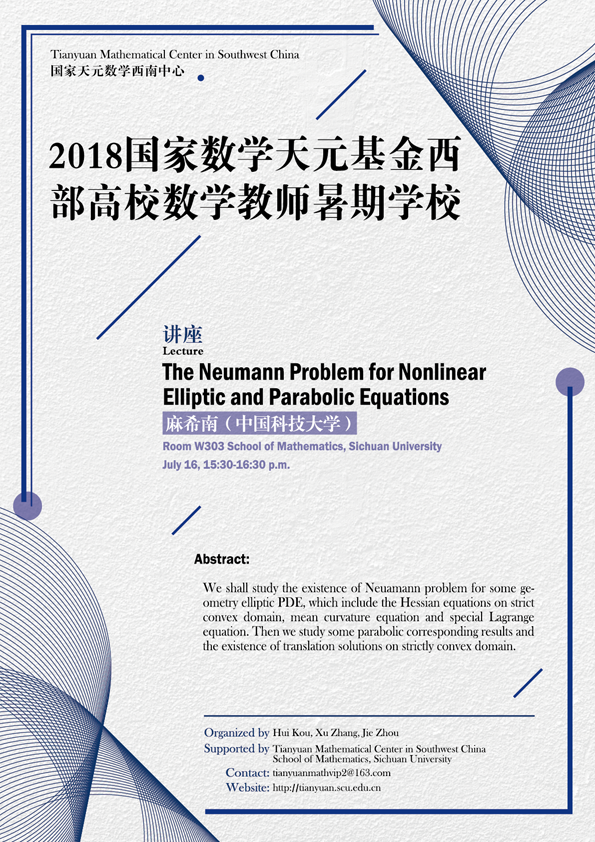The Neumann Problem for Nonlinear Elliptic and Parabolic Equations

#### W303  School of Mathematics, Sichuan University#### ABSTRACT

We shall study the existence of Neuamann problem for some geometry elliptic PDE, which include the Hessian equations on strict convex domain, mean curvature equation and special Lagrange equation. Then we study some parabolic corresponding results and the existence of translation solutions on strictly convex domain.

#### ORGANIZERS

Hui Kou (Sichuan University)

Xu Zhang (Sichuan University)

Jie Zhou (Sichuan University)

#### SUPPORTED BY

Tianyuan Mathematical Center in Southwest China

School of Mathematics, Sichuan University

#### VIDEO

• The Neumann Problem for Nonlinear Elliptic and Parabolic Equations
• 15:30 - 16:30, 2018-07-16
• 麻希南（中国科技大学）